TitleCollege Algebra
Tutorial 46: Logarithmic EquationsLearning Objectives

 After completing this tutorial, you should be able to: Solve logarithmic equations.Introduction

 In this tutorial I will step you through how to solve equations that have logarithmic expressions.  In these equations, you will notice that the variable that we are solving for is inside the log expressions.  We will rewrite the log equation exponentially using the definition of logs to help us get the x outside of the log.  If you need a review on the definition of log functions, feel free to go to Tutorial 43: Logarithmic Functions.  Ready, set, GO!!!!!Tutorial

 Solving a Logarithmic Equation of the Form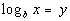Step 1:  Write as one log isolated on one side.

 Get your log on one side everything outside of the log on the other side of your equation using inverse operations.  Also use properties of logs to write it so that there is only one log.  If you need a review on properties of logs feel free to go to Tutorial 44: Logarithmic Properties.

 Step 2: Use the definition of logarithms to write in exponential form.

 A reminder that the definition of logarithms is the logarithmic function with base b, where b > 0 and b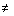0, and is defined asif and only if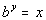. If you need a review on the definition of log functions, feel free to go to Tutorial 43: Logarithmic Functions.

 Step 3: Solve for x.

 Now that the variable is out of the log, solve for the variable using inverse operations to complete the problem.Example 1: Solve the logarithmic equation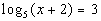.  Round your answer to two decimal places.

 This is already done for us in this problem.

 Step 2: Use the definition of logarithms to write in exponential form.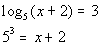*Rewrite in exponential form *Base = 5 and exponent = 3

 Step 3: Solve for x.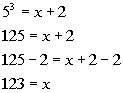*5 cubed is 125 *Solve for xExample 2: Solve the logarithmic equation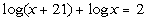.  Round your answer to two decimal places.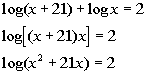*Use the product rule to write as one log *one log is isolated

 Step 2: Use the definition of logarithms to write in exponential form.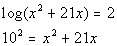*Rewrite in exponential form *Base = 10 and exponent = 2

 Remember that when there is no base written on a log, that means it is the common log, or log base 10.  If you need a review on common logs feel free to go to Tutorial 43: Logarithmic Functions.

 Step 3: Solve for x.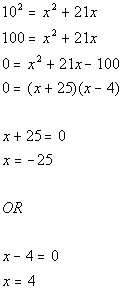*10 squared is 100 *Solve for x *Set 1st factor = 0          *Set 2nd factor = 0

 Since -25 would create a negative number inside both logs in this problem and we CANNOT take the log of a negative number, we will have to throw out x = -25 as one of our solutions.   Final answer: x = 4.Example 3: Solve the logarithmic equation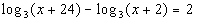.  Round your answer to two decimal places.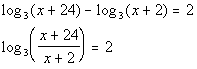*Use the quotient rule to write as one log *one log is isolated

 Step 2: Use the definition of logarithms to write in exponential form.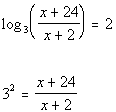*Rewrite in exponential form *Base = 3 and exponent = 2

 Step 3: Solve for x.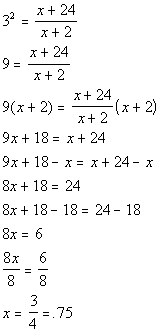*3 squared is 9 *Solve for x   *Multiply both sides by LCD of x + 2

 The equation that we had to solve in step 3 had a rational expression in it.  If you need a review on solving equations with rational expressions feel free to go to Tutorial 15: Equations with Rational Expressions.Example 4: Solve the logarithmic equation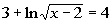.  Round your answer to two decimal places.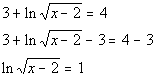*Inverse of add 3 is sub. 3 *one log is isolated

 Step 2: Use the definition of logarithms to write in exponential form.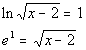*Rewrite in exponential form *Base = e and exponent = 1

 Remember that when you have ln, that means it is the natural log, or log base e.  If you need a review on natural logs feel free to go to Tutorial 43: Logarithmic Functions.

 Step 3: Solve for x.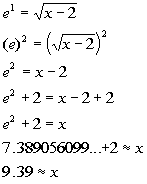*Square both sides to get rid of the radical *Solve for x   *Use the calculator to find e squared

 The equation that we had to solve in step 3 had a square root in it.  If you need a review on solving equations with radicals feel free to go to Tutorial 19: Radical Equations and Equations Involving Rational Exponents.Practice Problems

 These are practice problems to help bring you to the next level.  It will allow you to check and see if you have an understanding of these types of problems. Math works just like anything else, if you want to get good at it, then you need to practice it.  Even the best athletes and musicians had help along the way and lots of practice, practice, practice, to get good at their sport or instrument.  In fact there is no such thing as too much practice. To get the most out of these, you should work the problem out on your own and then check your answer by clicking on the link for the answer/discussion for that  problem.  At the link you will find the answer as well as any steps that went into finding that answer.Practice Problems 1a - 1d:  Solve the logarithmic equation.  Round your answer to two decimal places.

 1a.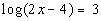(answer/discussion to 1a) 1b.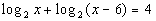(answer/discussion to 1b)

 1c.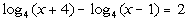(answer/discussion to 1c) 1d.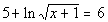(answer/discussion to 1d)Need Extra Help on these Topics?

The following are webpages that can assist you in the topics that were covered on this page.

 http://www.purplemath.com/modules/solvelog.htm This webpage will help you with solving logarithmic equations. http://www.sosmath.com/algebra/solve/solve8/s81/s81.html This webpage gives an example of solving a logarithmic equation.

Go to Get Help Outside the Classroom found in Tutorial 1: How to Succeed in a Math Class for some more suggestions.

Last revised on March 24, 2011 by Kim Seward.